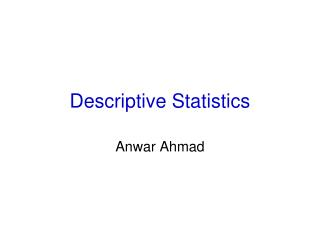DownloadDownload PresentationDescriptive Statistics

# Descriptive Statistics

Télécharger la présentation## Descriptive Statistics

- - - - - - - - - - - - - - - - - - - - - - - - - - - E N D - - - - - - - - - - - - - - - - - - - - - - - - - - -
##### Presentation Transcript

2. Central Tendency- Measure of location • Measures descriptive of a typical or representative value in a group of observations • It applies to groups rather than individuals

3. Arithmetic Mean • Simplest and obvious measure of central tendency • Simple average of the observations in the group, i.e. the value obtained by adding the observations together and dividing this sum by the number of observations in the group

4. Arithmetic Mean Example: 4,5,9,1,2 21/5 4.2

5. Median • The middle value in a set of observations ordered by size • Median income or median house price • 1,2,4,5,9 • 4 is the median

6. Mode • The most frequently occurring value in a set of observations.1,2,2,4,5,9 • 2 is the mode

7. Other Measures of Central Tendency • Midrange: The value midway between the smallest and largest values in the sample, that is, the arithmetic mean the largest and smallest values, the extremes. • 4,5,9,1,2 • (9+1)/2 • 5

8. Geometric Mean • The geometric mean of a set of observations is the nth root of their product. • Gm of 4 & 9 • Sqrt 4*9 • Sqrt 36 • 6

9. Harmonic Mean • The harmonic mean of a set of observations is the reciprocal (1/x) of the arithmetic mean of the reciprocals of the observations.

10. Harmonic Mean • Av. Velocity of car that traveled first 10 mi. at 30 mph; and the second 10 mi. at 60 mph. • Mean 30+60 /2 = 45 ? • Total distance by total time • 10+10 / 1/3 + 1/6 hr (1/2 hr) • 20/ ½ hr • Av. velocity 40 mph

11. Harmonic Mean • Harmonic mean • 2/ (1/30+1/60) = 40

12. Weighted Mean • When all observations do not have equal weight • Lab A 50 cultures, 25 positive, 50% • Lab B 80 cultures, 60 positive, 75% • Lab C 120 cultures, 30 positive, 25% = 150/3 =50% • WM = 50(50%)+80(75%)+120(25%) / 50+80+12 • 46%

13. Measure of Variability • 1,4,4,4,7 = 20 = 20/5 = 4 variation • 4,4,4,4,4 = 20 = 20/5 = 4 no variation • Same means, median, mode • 0 if no variation • Some + value, if there is a variation • Variation from the mean

14. Measure of Variability • Range • Variance • Standard Deviation

15. Range • Range is the simplest measure of spread or dispersion: • It is the difference between the largest and the smallest values. • The range can be a useful measure of spread because it is easily understood. • However, it is very sensitive to extreme scores since it is based on only two values.

16. Range • The range should almost never be used as the only measure of spread, but can be informative if used as a supplement to other measures of spread such as the standard deviation or variance

17. Variance • Squared deviation from the mean. • 1,4,4,4,7, mean 4 • (1-4), (4-4), (4-4), (4-4), (7-4) • -3, 0, 0, 0, 3 = 0 • -32, 0, 0, 0, 32 = 18/5 = 18/4 = 4.5

18. Variance • The variance describes the heterogeneity of a distribution and is calculated from a formula that involves every score in the distribution. It is typically symbolized by the letter s with a superscript "2". The formula is Variance, s2=sum (scores - mean)2/(n - 1) degree of freedom

19. Variance • The variance is a measure of how spread out a distribution is. It is computed as the average squared deviation of each number from its mean.

20. Standard deviation • The square root (the positive one) of the variance is known as the "standard deviation." It is symbolized by s with no superscript. • Sqrt 4.5 • 2.12

21. Summary Formulae